# 性能分析不一定得用 Profiler，复杂度分析也行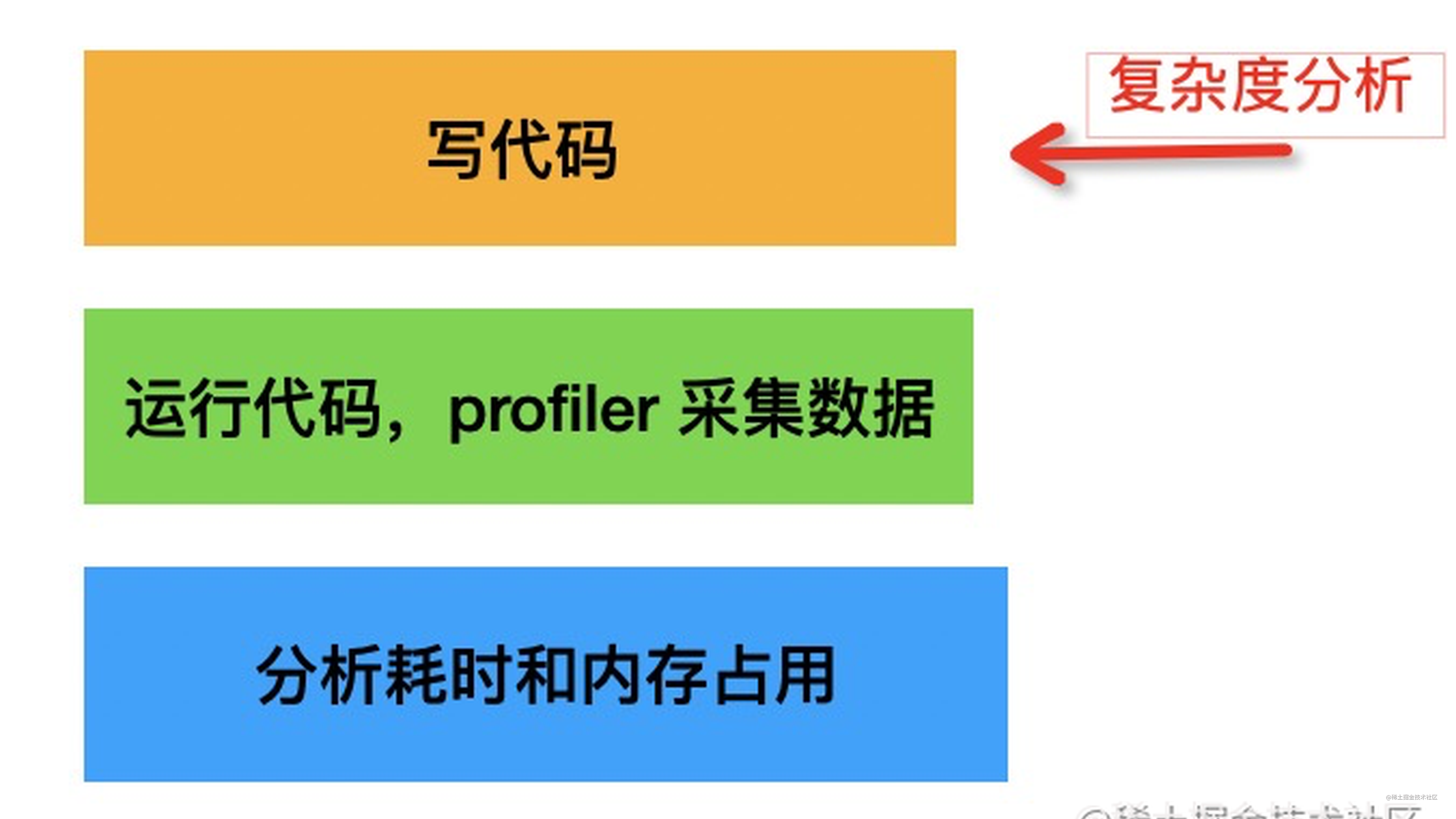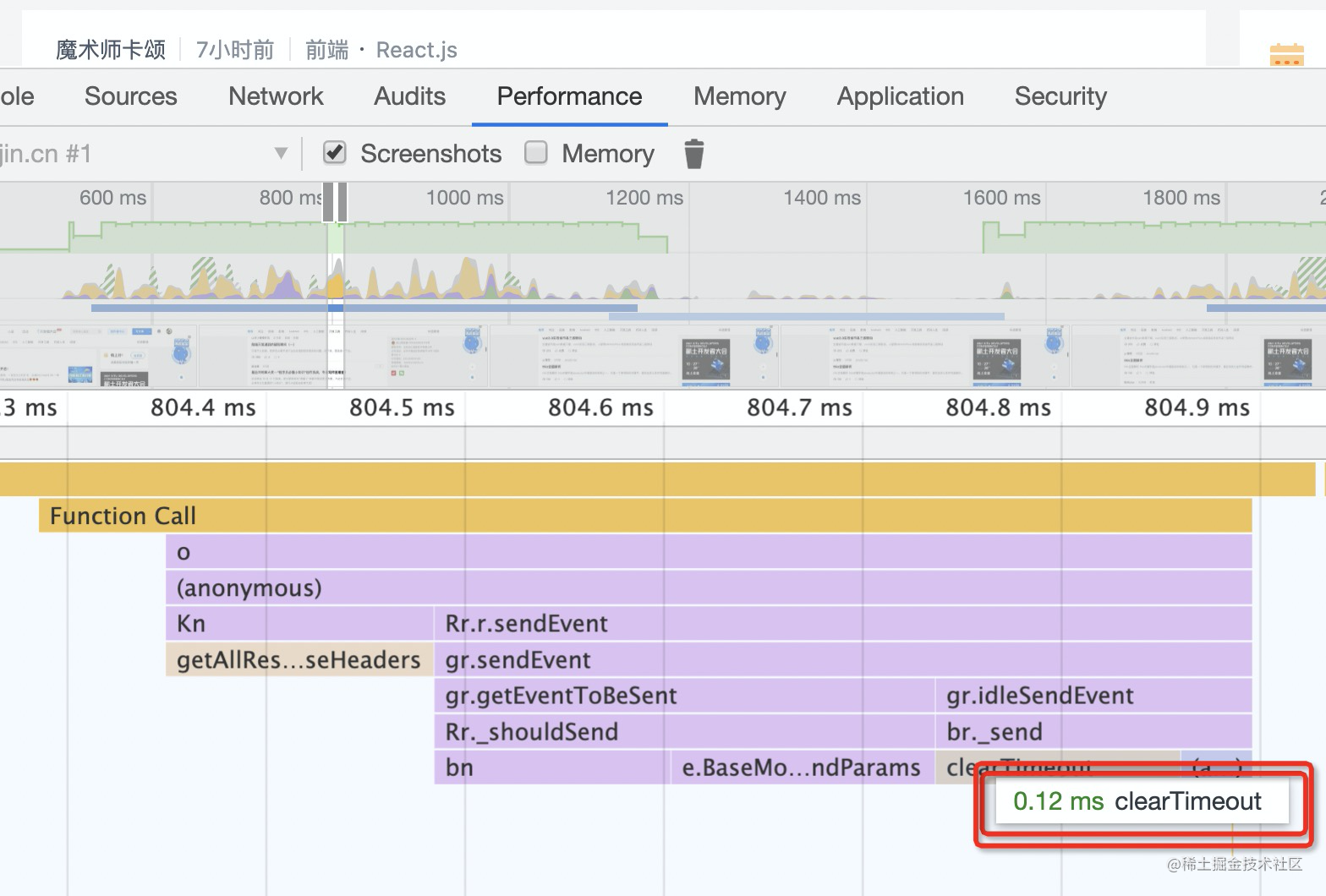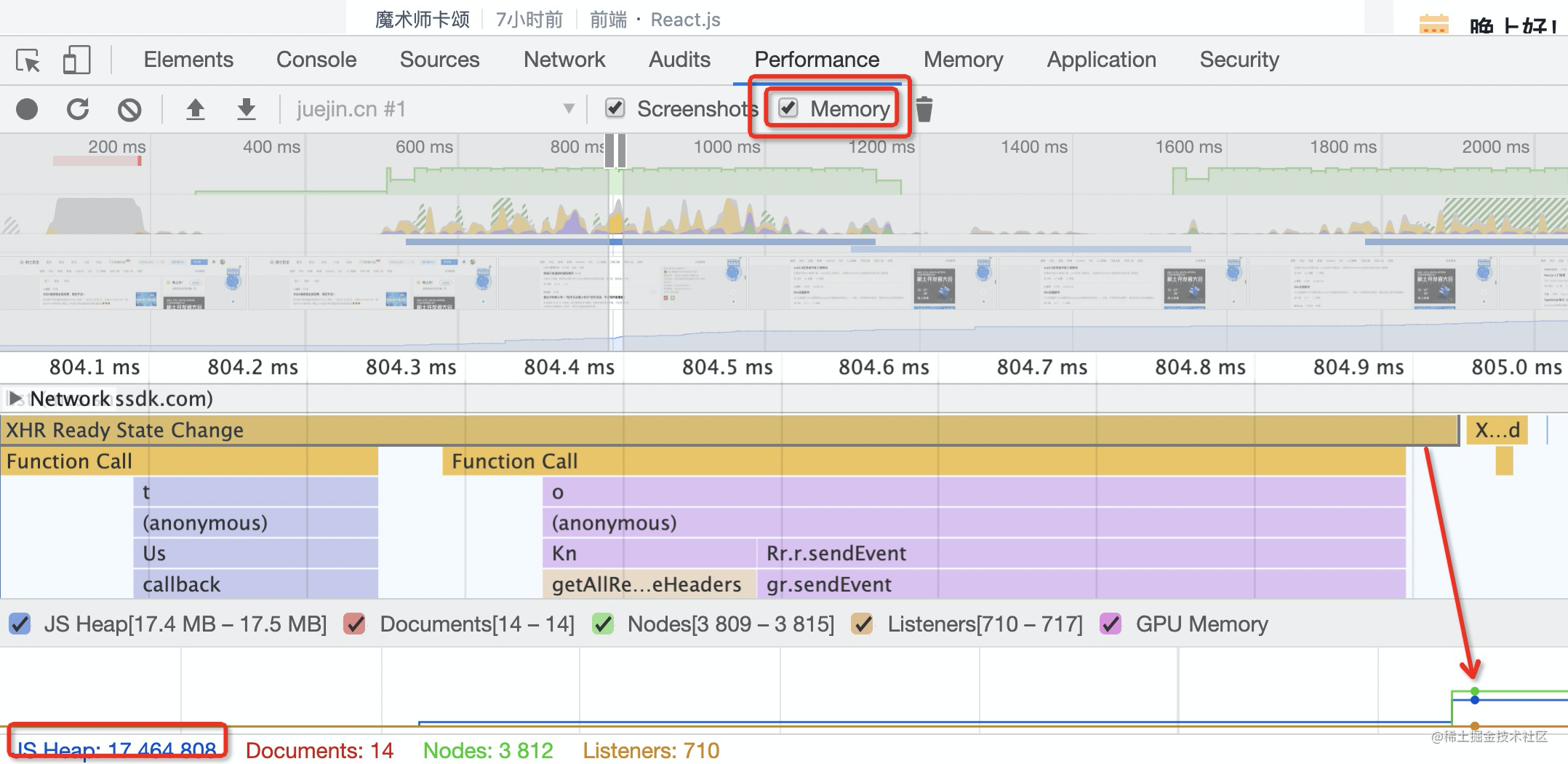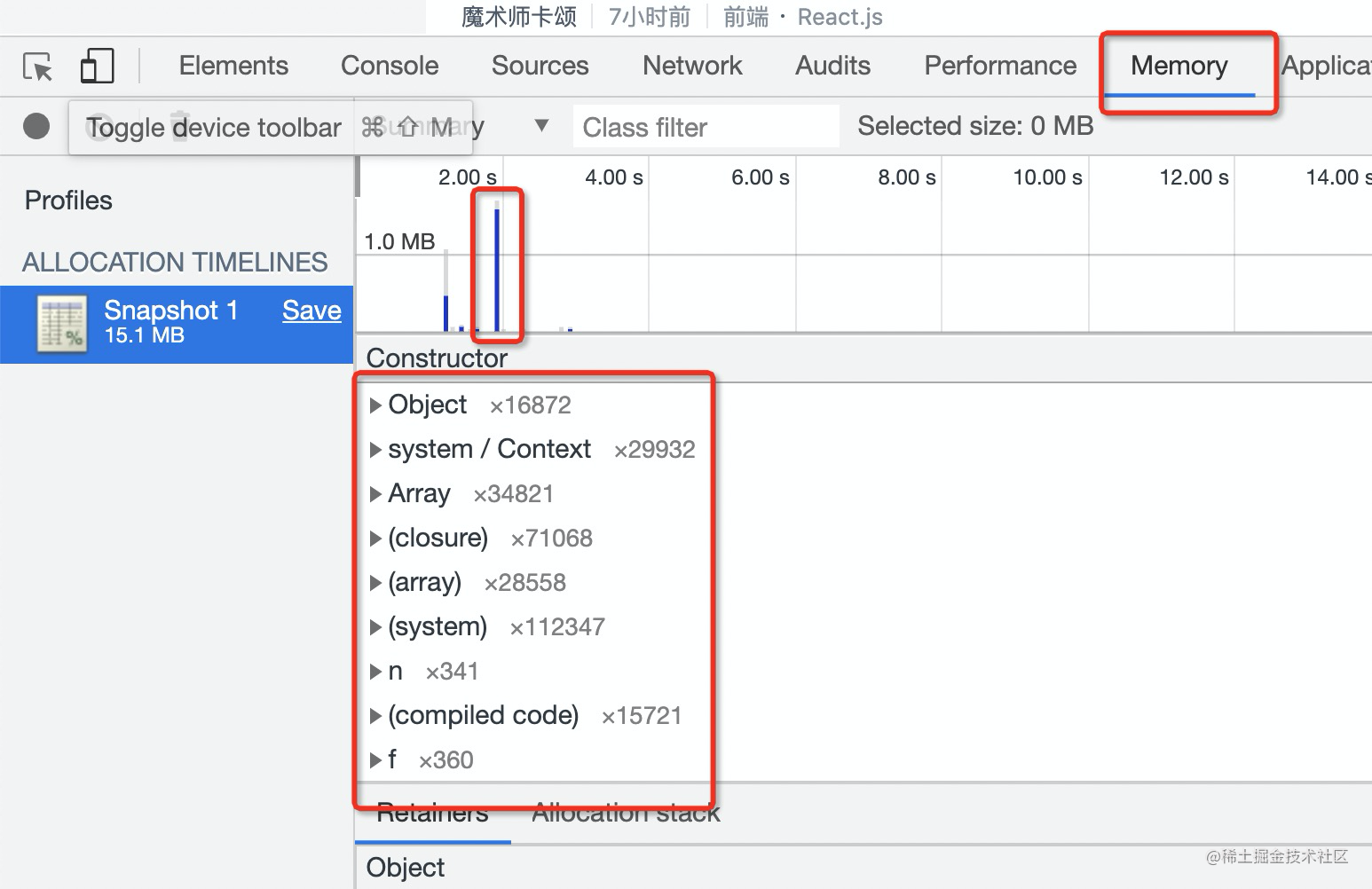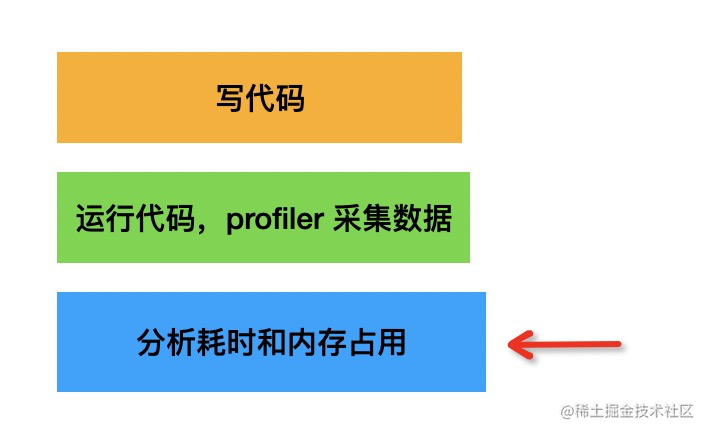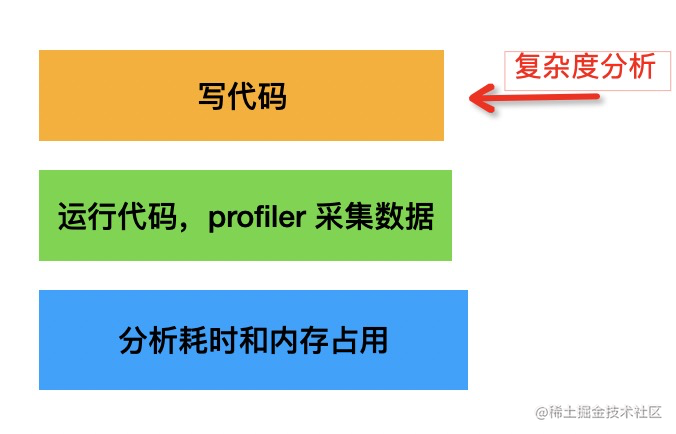## 复杂度分析的几个基础

### 什么是 1，什么是 n

``````const name = 'guang';

``````function calc(n) {
for(let i = 0; i < n; i ++) {
//...
}
}

### 渐进的时候，常数可省略

``````function func(n) {
const a = 1;
const b = 2;

for (let i = 0; i < n; i ++) {
//...
}
}

``````复杂度(func) = 2 + n

``````复杂度(func) =  O(n)

## 一些常见的时间复杂度

### O(n)

``````function func(n) {
const a = 1;
for(let i = 0; i < n; i ++) {
//...
}
}

### O(n^2) O(n^3)

``````function func(n) {
for(let i = 0; i < n; i ++) {
for(let j = 0; j < n; j ++) {
//...
}
}
}

### O(logn)

``````let n = 100;
let i = 1;
while(i < n){
i = i * 2
}

### o(2^n) o(3^n)

``````const fibnacci = function (n) {
if (n <= 1) return n;
return fibnacci(n - 1) + fibnacci(n - 2);
};

### O(n!)

``````function func(n) {
for(let i=0; i<n; i++) {
func(n-1);
}
}

## 时间复杂度的优劣对比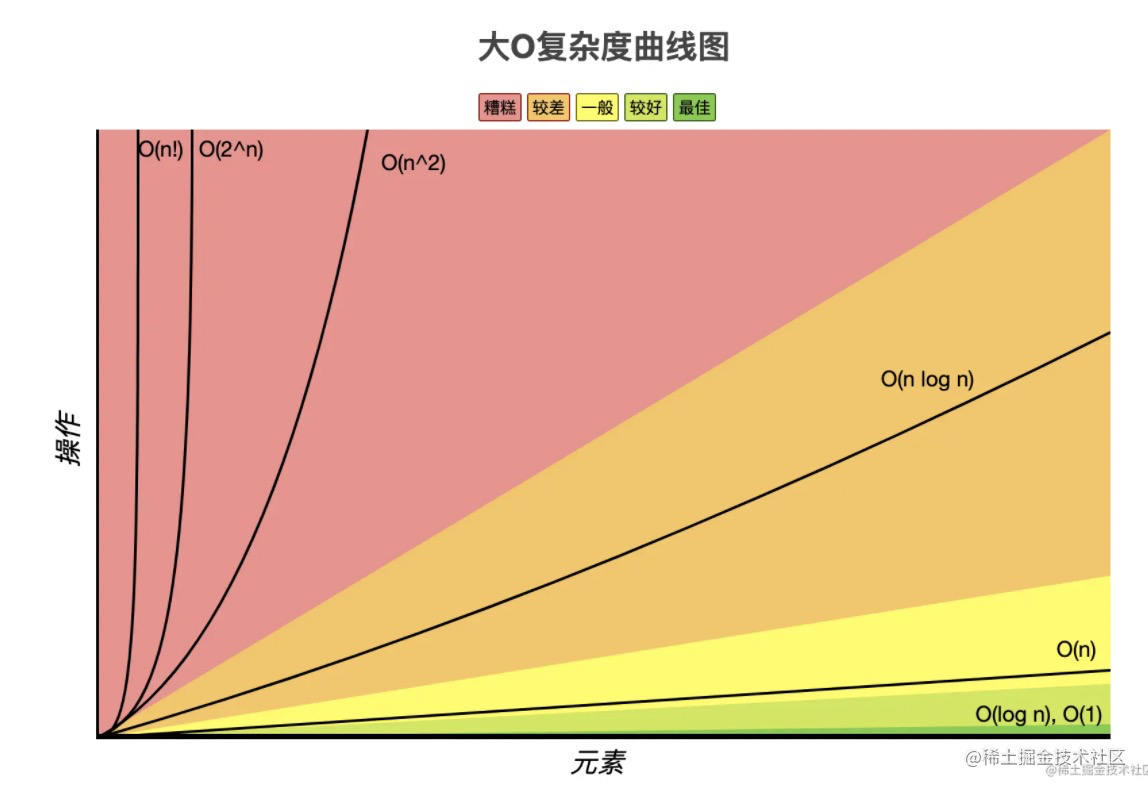• O(n!)、O(2^n) 的时间复杂度都是特别高的，是不好的，是要避免的。
• O(n)、O(nlogn)、O(logn) 的时间复杂度是比较低的，是好的，是要尽量采用的。

## 空间复杂度

``````function func(n) {
let arr = [];
for (let i = 0; i < n; i++) {
arr.push(i);
}
}

## 总结

O(2^n) 和 O(n!) 的复杂度都是随着 n 增加，复杂度急剧增加的，也就是耗时/内存占用会急剧增加，这样的代码很容易卡死，所以是不好的。